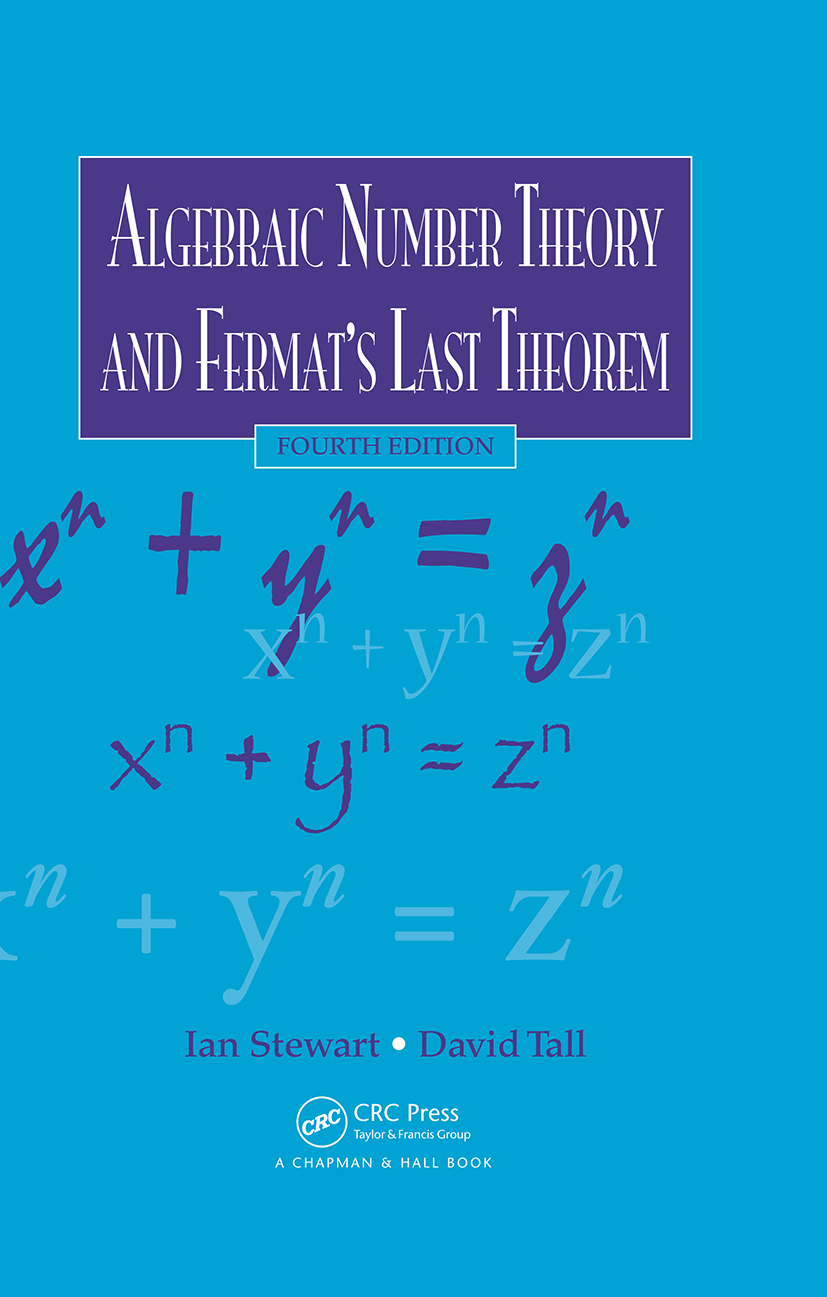# Algebraic Number Theory and Fermat's Last Theorem

## 4th Edition

Chapman and Hall/CRC

322 pages | 21 B/W Illus.

Hardback: 9781498738392
pub: 2015-10-13
SAVE ~\$16.79
\$83.95
\$67.16
x

FREE Standard Shipping!

### Description

Updated to reflect current research, Algebraic Number Theory and Fermat’s Last Theorem, Fourth Edition introduces fundamental ideas of algebraic numbers and explores one of the most intriguing stories in the history of mathematics—the quest for a proof of Fermat’s Last Theorem. The authors use this celebrated theorem to motivate a general study of the theory of algebraic numbers from a relatively concrete point of view. Students will see how Wiles’s proof of Fermat’s Last Theorem opened many new areas for future work.

New to the Fourth Edition

• Provides up-to-date information on unique prime factorization for real quadratic number fields, especially Harper’s proof that Z(√14) is Euclidean
• Presents an important new result: Mihăilescu’s proof of the Catalan conjecture of 1844
• Revises and expands one chapter into two, covering classical ideas about modular functions and highlighting the new ideas of Frey, Wiles, and others that led to the long-sought proof of Fermat’s Last Theorem
• Improves and updates the index, figures, bibliography, further reading list, and historical remarks

Written by preeminent mathematicians Ian Stewart and David Tall, this text continues to teach students how to extend properties of natural numbers to more general number structures, including algebraic number fields and their rings of algebraic integers. It also explains how basic notions from the theory of algebraic numbers can be used to solve problems in number theory.

### Reviews

"It is the discussion of [Fermat’s Last Theorem], I think, that sets this book apart from others — there are a number of other texts that introduce algebraic number theory, but I don’t know of any others that combine that material with the kind of detailed exposition of FLT that is found here…To summarize and conclude: this is an interesting and attractive book. It would make an attractive text for an early graduate course on algebraic number theory, as well as a nice source of information for people interested in FLT, and especially its connections with algebraic numbers."

Dr. Mark Hunacek, MAA Reviews, June 2016

Praise for Previous Editions

"The book remains, as before, an extremely attractive introduction to algebraic number theory from the ideal-theoretic perspective."

—Andrew Bremner, Mathematical Reviews, February 2003

Algebraic Methods

Algebraic Background

Rings and Fields

Factorization of Polynomials

Field Extensions

Symmetric Polynomials

Modules

Free Abelian Groups

Algebraic Numbers

Algebraic Numbers

Conjugates and Discriminants

Algebraic Integers

Integral Bases

Norms and Traces

Rings of Integers

Cyclotomic Fields

Factorization into Irreducibles

Historical Background

Trivial Factorizations

Factorization into Irreducibles

Examples of Non-Unique Factorization into Irreducibles

Prime Factorization

Euclidean Domains

Consequences of Unique Factorization

The Ramanujan–Nagell Theorem

Ideals

Historical Background

Prime Factorization of Ideals

The Norm of an Ideal

Nonunique Factorization in Cyclotomic Fields

Geometric Methods

Lattices

Lattices

The Quotient Torus

Minkowski's Theorem

Minkowski's Theorem

The Two-Squares Theorem

The Four-Squares Theorem

Geometric Representation of Algebraic Numbers

The Space Lst

Class-Group and Class-Number

The Class-Group

An Existence Theorem

Finiteness of the Class-Group

How to Make an Ideal Principal

Unique Factorization of Elements in an Extension Ring

Number-Theoretic Applications

Computational Methods

Factorization of a Rational Prime

Minkowski Constants

Some Class-Number Calculations

Table of Class-Numbers

Kummer's Special Case of Fermat's Last Theorem

Some History

Elementary Considerations

Kummer's Lemma

Kummer's Theorem

Regular Primes

The Path to the Final Breakthrough

The Wolfskehl Prize

Other Directions

Modular Functions and Elliptic Curves

The Taniyama–Shimura–Weil Conjecture

Frey's Elliptic Equation

The Amateur Who Became a Model Professional

Technical Hitch

Flash of Inspiration

Elliptic Curves

Review of Conics

Projective Space

Rational Conics and the Pythagorean Equation

Elliptic Curves

The Tangent/Secant Process

Group Structure on an Elliptic Curve

Applications to Diophantine Equations

Elliptic Functions

Trigonometry Meets Diophantus

Elliptic Functions

Legendre and Weierstrass

Modular Functions

Wiles's Strategy and Recent Developments

The Frey Elliptic Curve

The Taniyama–Shimura–Weil Conjecture

Sketch Proof of Fermat's Last Theorem

Recent Developments

Appendices

The Units of Zm

Dirichlet’s Units Theorem

Introduction

Logarithmic Space

Embedding the Unit Group in Logarithmic Space

Dirichlet's Theorem

Bibliography

Index

Exercises appear at the end of each chapter.